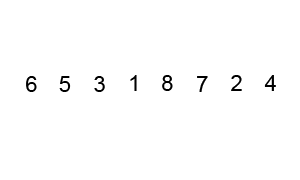# Bubble sort facts for kids

Kids Encyclopedia Facts

Bubble sort is a simple sorting algorithm. It is simple to understand, so it is usually taught to new students. It is not as efficient as some other sorting algorithms.

Bubble sort's name comes from the fact that each item in the list “bubbles” up to where it should go, like bubbles in water.

## AlgorithmAn example of bubble sort. Starting from the beginning of the list, compare the next pair. Swap their positions if they are not in the right order (the second one in the pair is smaller than the first one in the pair). After each iteration, one less element (the last one) is needed to be compared until there are no more elements left to be compared.

The algorithm compares pairs of elements in a list. The elements the make up the pairs are next to each other. Starting at one end of the list, the two elements in each pair are compared to each other in order. That means for example, the first and second element are compared, then the second and third element, and then the third and fourth, and so on. If the elements in the current pair are out of order, then the two elements switch places. This process – of comparing two elements – is done over and over again, until the whole list is sorted. The list is sorted, when there are no more pairs that have to be swapped.

In the best case scenario, where the list was already sorted before running the algorithm, the algorithm's complexity is O(n) (Big O notation). In the worst case, where the list starts off as being sorted in reverse, O(n²).

## Implementation

In an imperative programming language, bubble sort can be implemented by using a flag variable and looping through the array's elements:

1. Set the flag `sorted`.
2. Starting at one end, consider every neighbored pair of elements in a vector one after another (in their order).
3. If a pair's elements are out of order, swap them, and clear the flag `sorted`.
4. Repeat the previous steps until `sorted` remains set.

Alternatively, since the greatest value ascends to the highest index within the first iteration and then has reached its final right position, two for-loops nested into one another sort the vector, too:

```for top ≔ high(vector)−1 downto low(vector) do
for current ≔ low(vector) to top do
if vector[current] > vector[current+1] then
exchange(vector, current, current+1)
```

## Images for kidsBubble sort Facts for Kids. Kiddle Encyclopedia.